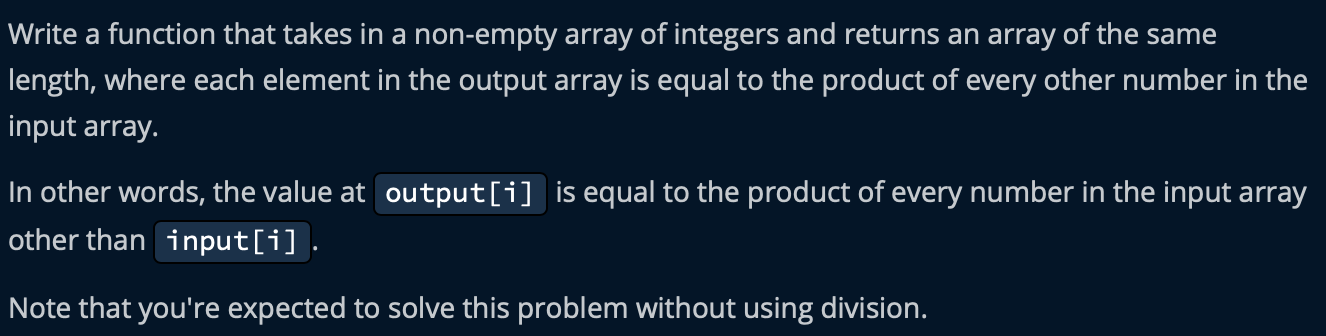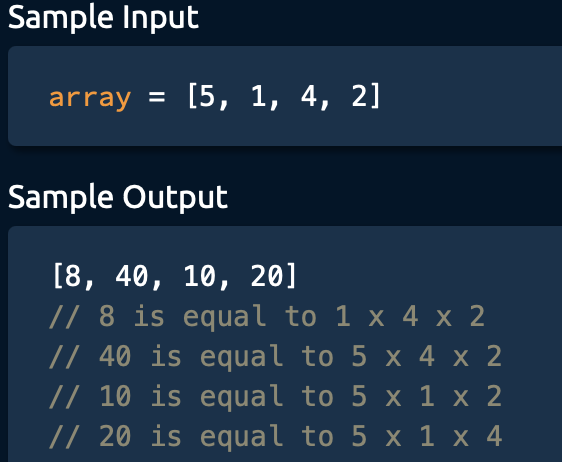5G时代下一个聚合的编程学习网

# Array of products

## Problem Statement## Sample input## Analysis

### code. time complexity: O(N^2).  space complexity: O(N)

```def arrayOfProducts(array):
product = [1 for i in range(len(array))]
for i in range(len(array)):
res = 1
for j in range(len(array)):
if i != j:
res *= array[j]
product[i] = res
return product     ```

### code.  time complexity: O(N).  space complexity: O(N)

```def arrayOfProducts(array):
left = [1 for i in range(len(array))]
right = [1 for i in range(len(array))]
res = [1 for i in range(len(array))]

leftProduct = 1
rightProduct = 1
for i in range(len(array)):
left[i] = leftProduct
leftProduct *= array[i]

for i in reversed(range(len(array))):
right[i] = rightProduct
rightProduct *= array[i]

for i in range(len(array)):
res[i] = right[i] * left[i]
return res```

### code.  time complexity: O(N).  space complexity: O(N)

```def arrayOfProducts(array):
product = [1 for i in range(len(array))]

leftProduct = 1
rightProduct = 1

for i in range(len(array)):
product[i] = leftProduct
leftProduct *= array[i]

for i in reversed(range(len(array))):
product[i] *= rightProduct
rightProduct *= array[i]

return product```